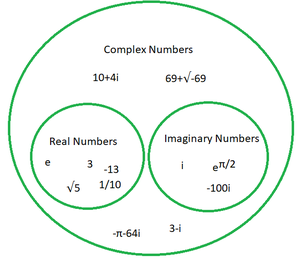# Find the real and imaginary parts of (2+2i)ei/2

• Last Updated : 16 Dec, 2021

Numbers that are capable of getting plotted on a number line are called real number. They include integers, whether positive or negative, rational numbers as well as irrational numbers, whether in their fraction form or decimal form.

The polar opposite of real numbers are imaginary numbers. Simply stated, such numbers as are not a part of the number line are called imaginary numbers. A number which yields a negative result when multiplied with itself is termed as an imaginary number. An imaginary number, when written without using the radical expression can be written as a real number, multiplied by iota, depicted i, which is an imaginary unit and iota (i) = √-1. Hence, √-9 can be written as= 3i.

### Complex Numbers

When a real and an imaginary number is conjoined with a mathematical operator between them, the resultant number is termed as a complex number. In other words, a complex number is partly real and partly imaginary, the real part being any decimal, fraction, logarithmic or exponential or radical number and the imaginary part depicted with i.

A complex number is basically of the form z = a + ib, where a and b are real numbers. Thus in order to conjure up a complex number, all that one needs is two real numbers and the imaginary part, i, conjoined with a mathematical operator in between. Examples,

• 12 + 420i is a complex number, where 12 is the real part and 420i depicts the imaginary part.
• 8e2 + 69i  is a complex number, where 8e2 is the real part and 69i is the imaginary part.
• √(22) – 162i is a complex number, where √(22) is the real part and 162i is the imaginary part.### Find the real and imaginary parts of (2 + 2 i)ei/2.

Solution:

(2 + 2i) ei/2 = (2 + 2i) (cos1/2 + isin1/2)

cos 0.5 and sin 0.5 are in radians. Therefore,

= (2 + 2i) ( 0.877 + i0.479)

= (1.754 + 1.754i + 0.958i – 0.958)

= (0.796 + i2.712)

Thus, the real part = 0.796 and imaginary part = 2.712.

### Similar Problems

Question 1: Find the real and imaginary parts of ez if z = x + iy.

Solution:

ez = ex + iy

= ex(cosy + isiny)

= ex cos y + ex isiny

Hence the real part = ex cos y and the imaginary part = ex isiny.

Question 2: Find the real and imaginary parts of 3i20 – i19.

Solution:

Clearly, i20 = 1 and i19 = i.

So, the expression becomes 3(1) – i = 3 – i

Hence the real part = 3 and the imaginary part = 1.

Question 3: Find the real and imaginary parts of the number q if q ∈ R.

Solution:

q ∈ R, q is a real number, implying that it does not have any imaginary part. Alternatively one can say that the coefficient of i is zero.

Hence the real part and the imaginary part of q for all q ∈ R are q and zero respectively.

Question 4: Find the real and imaginary parts of 10i100 + 2i99.

Solution:

Clearly, i100 = 1 and i99 = -i

So, the expression becomes 10(1) + 2(-i) = 10 – 2i

Hence the real part = 10 and the imaginary part = 2.

Question 5: Find the real and imaginary parts of e-2 + i12.

Solution:

A complex number is usually written in the form z = a + ib, where a depicts the real part and ib or bi would be the imaginary constituent.

Real part ⇢ e-2 = 1/ e2 and imaginary part = 12i.

My Personal Notes arrow_drop_up
Recommended Articles
Page :